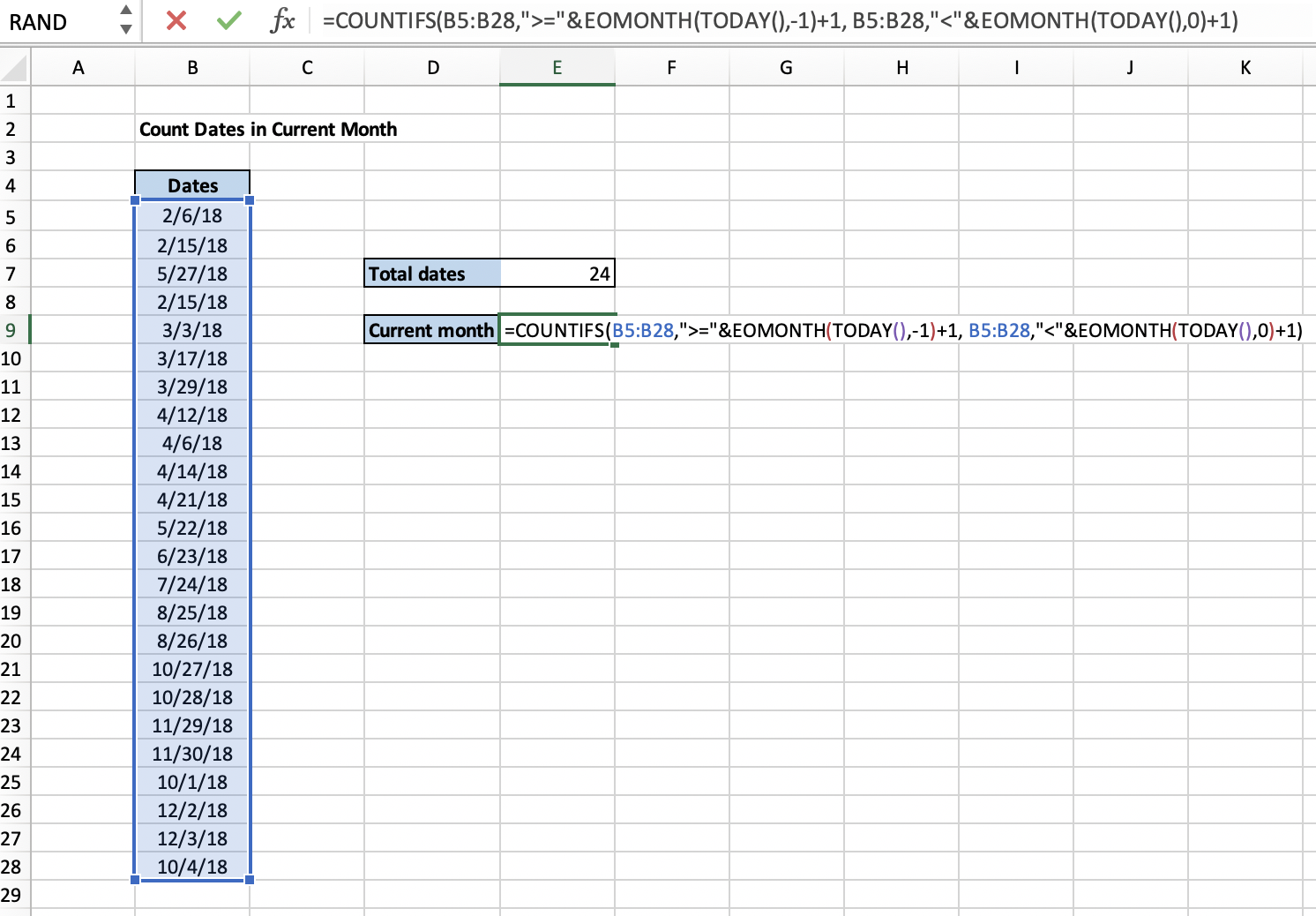Get instant live expert help with Excel or Google Sheets“My Excelchat expert helped me in less than 20 minutes, saving me what would have been 5 hours of work!”

#### Post your problem and you’ll get expert help in seconds.

Your message must be at least 40 characters
Our professional experts are available now. Your privacy is guaranteed.

# How to Count the Dates in the Current Month in Excel

Counting dates in the current month are crucial especially if you are running a project with a predetermined time frame. However, counting manually can be tiresome and not to mention possible errors. But you can avoid all these hustles by using COUNTIFS OR SUMPRODUCT function.

## How To Count Dates In Current Month

For the COUNTIFS function, here is the formula:

### Generic Formula:

`=COUNTIFS(rng,">="&EOMONTH(TODAY(),-1)+1,rng,"<"&EOMONTH(TODAY(),0)+1)`

…where “rng” dates range in the excel sheet. TODAY supplies the current date to the formula while EOMONTH provides actual dates.  Finally, COUNTIF will do the count current month dates.

### Example:

Check out how counting dates in the current month have been done in this example:Figure 1. Example 1 of COUNTIFS Function

In this screenshot, the formula in the highlighted cell (E9) is:

`=COUNTIFS(B5:B28,">="&EOMONTH(TODAY(),-1)+1, B5:B28,"<"&EOMONTH(TODAY(),0)+1)`

### How the Formula Works

The COUNTIF, which is the core function, count dates in the range and tests whether they meet the requirement, i.e., greater than or equal to the first day month of interest (current month) and less than the 1st day of the next month. EOMONTH creates both dates using the current dates which are a return of the TODAY function.

This is the formula that gets the first day of the month:

`=EOMONTH(TODAY(),-1)+1`

….. the function (EOMONTH) works by returning the previous month’s last day and adding 1 and that gives the first day of the current month.

### For the current month’s last date, here is the formula:

`EOMONTH(TODAY(),0)+1)`

That is how this formula counts dates in the current month. For this example, the count is 4

### SUMPRODUCT Alternative

This is another alternative that offers a direct solution. In addition, you can get dates for previous and even next month with it. The formula looks like this:

`=SUMPRODUCT(N(TEXT(EOMONTH(TODAY(),-1),"mmyy")=TEXT(rng,"mmyy")))`

`= SUMPRODUCT(N(TEXT(EOMONTH(TODAY(),"mmyy")= TEXT(rng,"mmyy")))`

`= SUMPRODUCT(N(TEXT(EOMONTH(TODAY(),1),"mmyy")= TEXT(rng,"mmyy")))`

To solve the problem, a chain of month and year returned by the TEXT function is compared with an array inside SUMPRODUCT. The result is an array of TRUE/FALSE where the TRUE values are dates for a month you are looking for.

The N function is responsible for changing TRUE/FALSE values to 1s and 0s for final tally by SUMPRODUCT.

Still need some help with Excel formatting or have other questions about Excel? Connect with a live Excel expert here for some 1 on 1 help. Your first session is always free.

### Did this post not answer your question? Get a solution from connecting with the expert.Another blog reader asked this question today on Excelchat:
Solution examplescan you teach me the steps to create a nested if function, and to nest an AND function inside of an IF function?
Solved by A. Q. in 22 minsI want to display a range of cells if they meet a specific criteria. I want to display a name IF it does not equal one of three options AND there are any numbers contained in a range of cells
Solved by G. F. in 60 minshow to make a format that if multiple cells have number contents its value is 1 in the last cell
Solved by C. C. in 28 minsI need to find out if the 2018 yearly sales goals were met if the yearly sales were \$25,000 or more using an IF logical function and to set the formula to return a value of YES if met, and NO if not
Solved by A. A. in 18 minsI'm looking for a formula or format for if there is data in c4 on a sheet... It adds extra information underneath
Solved by X. E. in 20 mins## Subscribe to Excelchat.coAnother blog reader asked this question today on Excelchat: